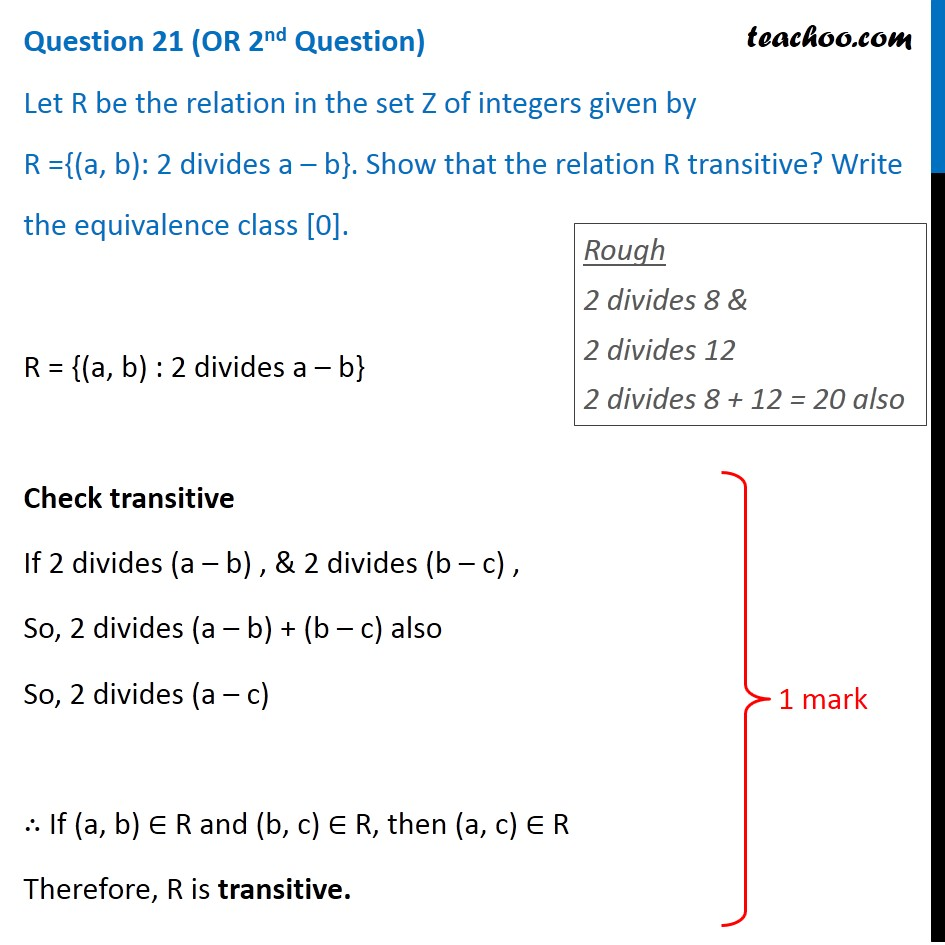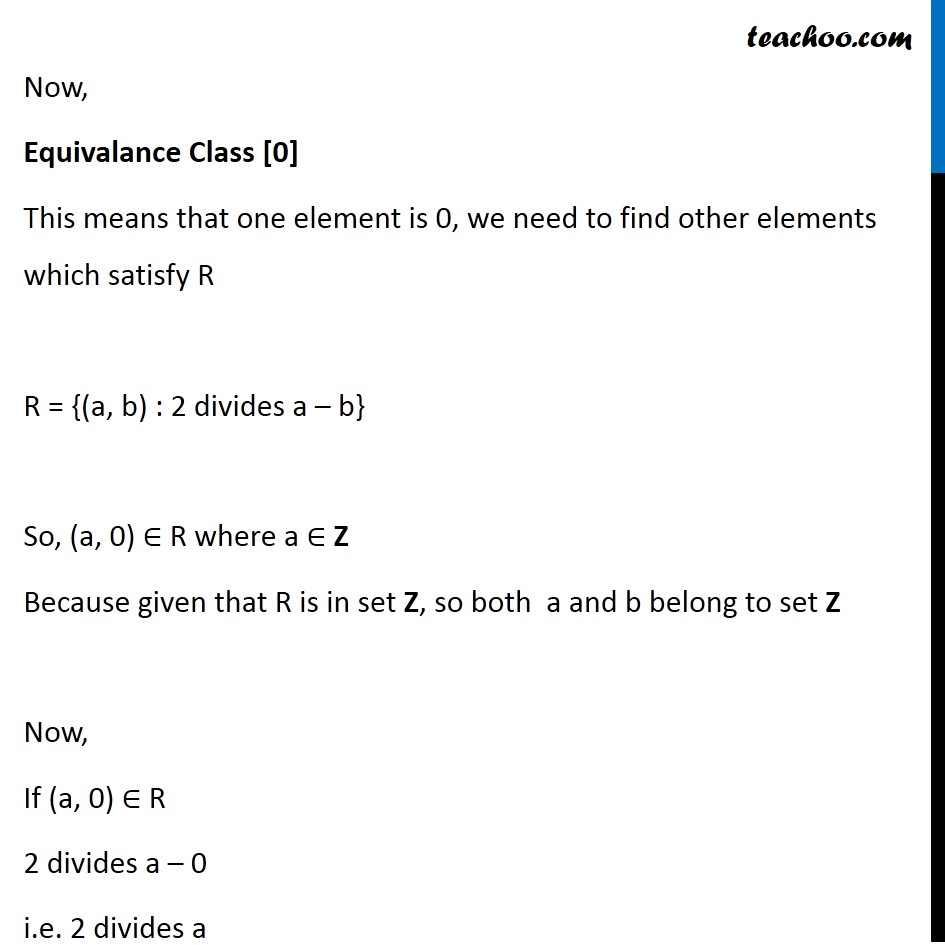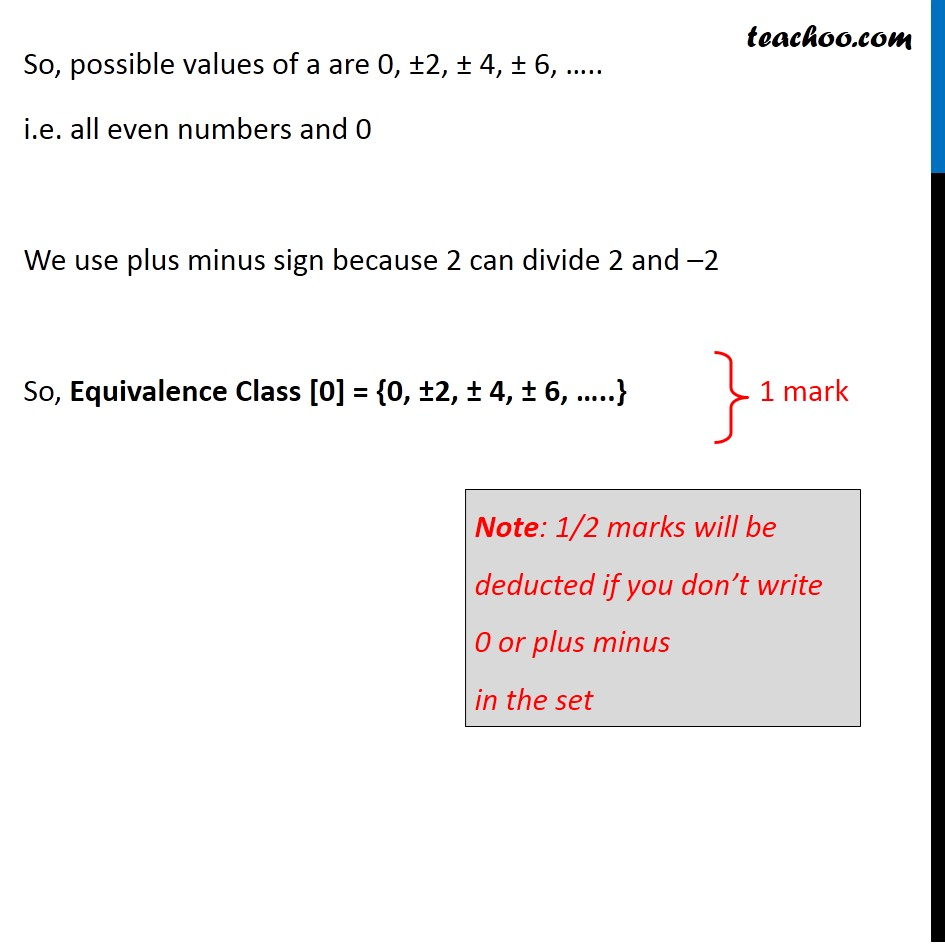CBSE Class 12 Sample Paper for 2020 Boards

Class 12
Solutions of Sample Papers and Past Year Papers - for Class 12 Boards

## R = {(a, b): 2 divides a – b}. Show that the relation R transitive? Write the equivalence class .Note : - This question is a part of Example 5 of NCERT – Chapter 1 Class 12

Learn in your speed, with individual attention - Teachoo Maths 1-on-1 Class

### Transcript

Question 21 (OR 2nd Question) Let R be the relation in the set Z of integers given by R ={(a, b): 2 divides a – b}. Show that the relation R transitive? Write the equivalence class . R = {(a, b) : 2 divides a – b} Check transitive If 2 divides (a – b) , & 2 divides (b – c) , So, 2 divides (a – b) + (b – c) also So, 2 divides (a – c) ∴ If (a, b) ∈ R and (b, c) ∈ R, then (a, c) ∈ R Therefore, R is transitive. Rough 2 divides 8 & 2 divides 12 2 divides 8 + 12 = 20 also Now, Equivalance Class  This means that one element is 0, we need to find other elements which satisfy R R = {(a, b) : 2 divides a – b} So, (a, 0) ∈ R where a ∈ Z Because given that R is in set Z, so both a and b belong to set Z Now, If (a, 0) ∈ R 2 divides a – 0 i.e. 2 divides a So, possible values of a are 0, ±2, ± 4, ± 6, ….. i.e. all even numbers and 0 We use plus minus sign because 2 can divide 2 and –2 So, Equivalence Class  = {0, ±2, ± 4, ± 6, …..} Note: 1/2 marks will be deducted if you don’t write 0 or plus minus in the set